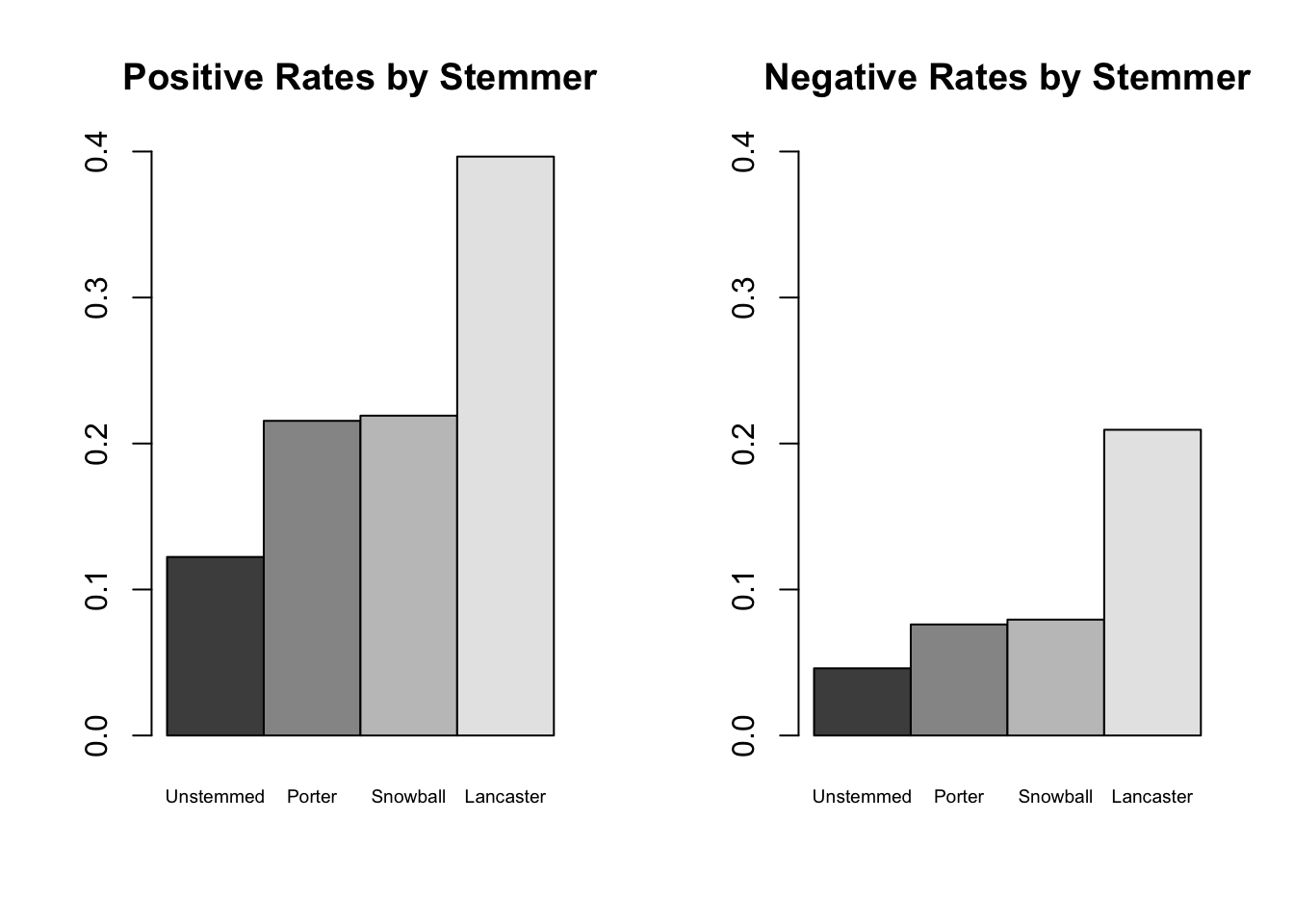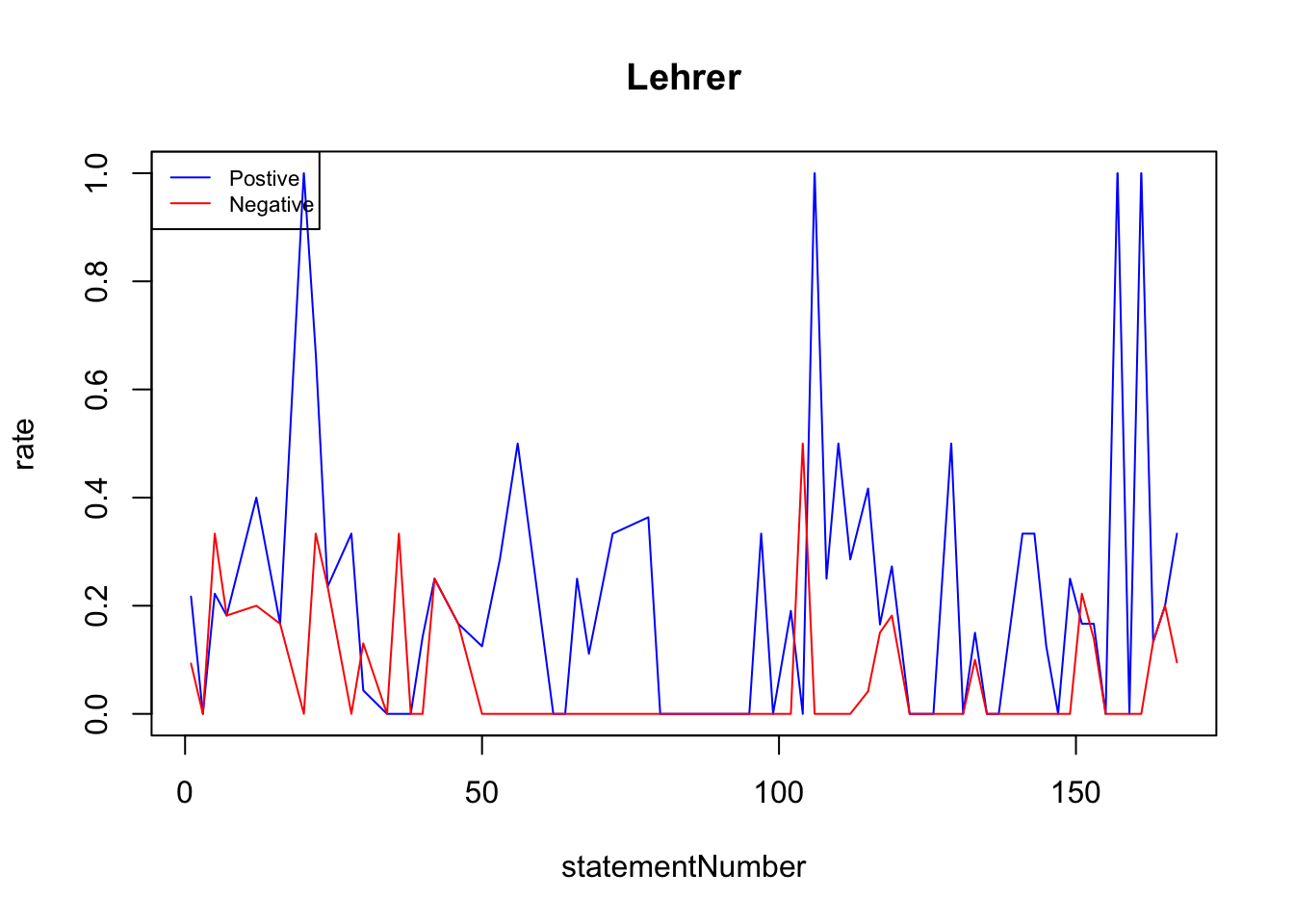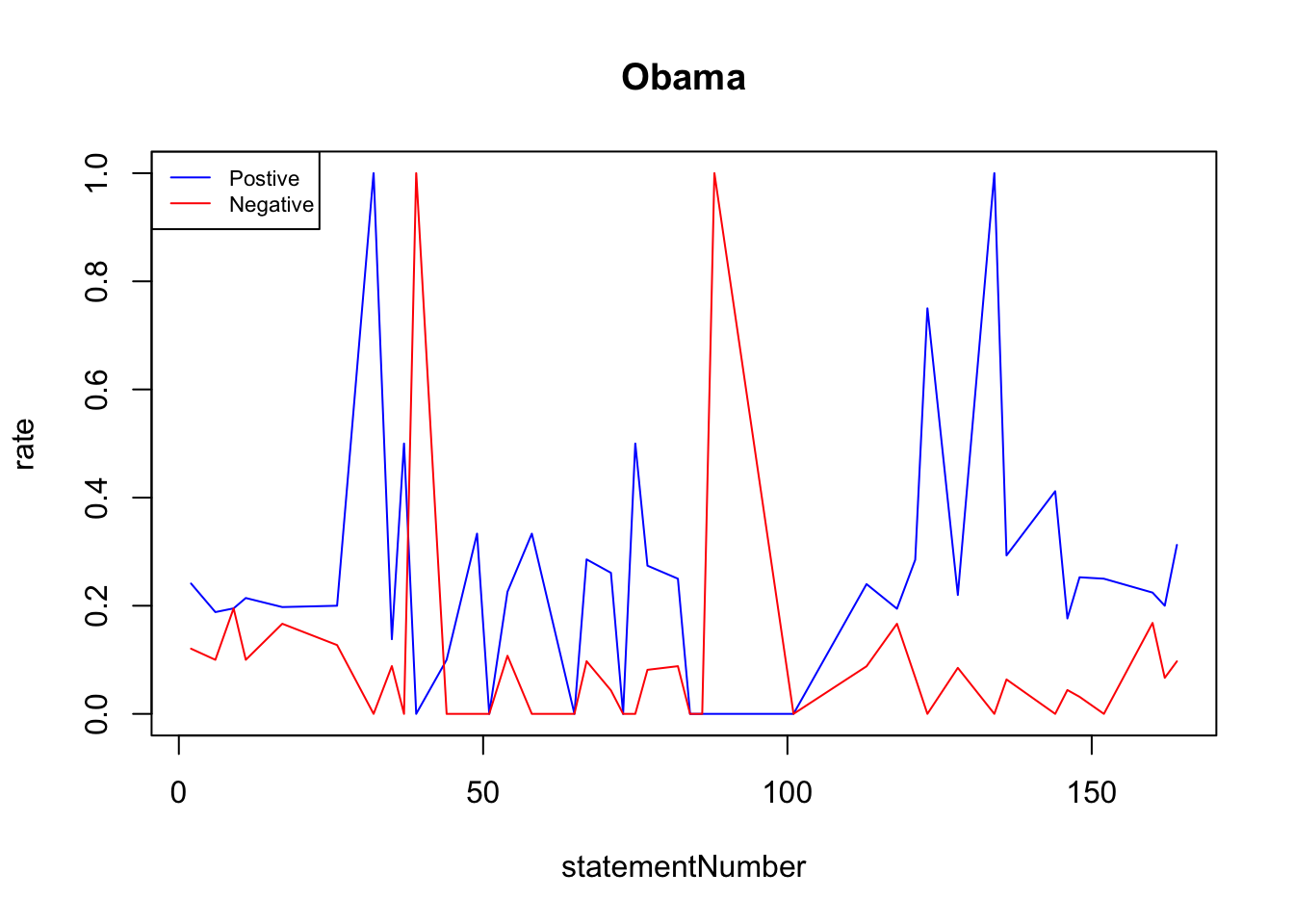### Problem 1

Preprocessing data with python.

``````
import os, re, csv, urllib2, nltk
from bs4 import BeautifulSoup

#set working directory
os.chdir("/Users/franceszlotnick/Dropbox/TextAsData/ProblemSets/PS2/")

#### Problem 1 ###################
#read in data
with open("Debate1.html", "r") as f:
text = f.read()

#create soup object, select <p> tags
soup = BeautifulSoup(text)
ps = soup.find_all("p")

#drop elements tags from before and after debate starts/ends.
ps = ps[6:477]

#create list of statements. Loop over <p> tags; if there is a label, append statement to
#list if the label is different from the previous label, otherwise append to previous
#statement. if no label, check if it is (APPLAUSE) or (CROSSTALK). If not, concatenate it onto
#the previous statement. Note that any strategy at this stage is likely to miss some statements
#because of inconsistent HTML formatting in our data.
statements = []
prev = ""

for tag in ps:
stmt= tag.get_text()
m = re.search('^[A-Z]+:', stmt)
if m:
if m.group() != prev:
statements.append(stmt)
prev = m.group(0)
else:
last = statements.pop()
statements.append(last + " " + stmt)
else:
if re.search('\([A-Z]+\)', stmt) == None:
last = statements.pop()
statements.append(last + " " + stmt)

######## Problem 2 ################
#import stemmers
porter = nltk.stem.PorterStemmer()
snowball = nltk.stem.SnowballStemmer('english')
lancaster = nltk.stem.LancasterStemmer()

#import dictionaries
pos = urllib2.urlopen("http://www.unc.edu/~ncaren/haphazard/positive.txt").read().split("\n")
neg = urllib2.urlopen("http://www.unc.edu/~ncaren/haphazard/negative.txt").read().split("\n")
stop_words = urllib2.urlopen('http://jmlr.org/papers/volume5/lewis04a/a11-smart-stop-list/english.stop').read().split('\n')

#create sets of dictionaries.
#note: we use sets because the dictionaries, especially once stemmed, contain many duplicates, and we only need
#to test for membership. sets are the most efficient data strcuture for this task. Note the set comprehension
#syntax, which is the same as list comprehension but surrounded by curly brackets instead of square brackets.

posPorter = {porter.stem(w) for w in pos}
posSnowball = {snowball.stem(w) for w in pos}
posLancaster = {lancaster.stem(w) for w in pos}
negPorter = {porter.stem(w) for w in neg}
negSnowball = {snowball.stem(w) for w in neg}
negLancaster = {lancaster.stem(w) for w in neg}
stop_words = set(stop_words)
pos = set(pos)
neg = set(neg)

#loop over statements list to create dictionary with each statement as a key with
# attributes we want as nested dictionaries

obs = {}
count = 1
for i in statements:
print "count = " + str(count)
obs[i] = {}
obs[i]["statementNumber"] = count
#record first all caps word in statement as speaker
obs[i]["speaker"] = re.search('^[A-Z]+', i).group()
#remove punctuation, capitalization, and tokenize statement
words = re.sub("\W", " ", i)
words = words.lower()
words = nltk.word_tokenize(words)
#find number of unstemmed words present in each dictionary
obs[i]["numNonStop"] = len([x for x in words if x not in stop_words])
obs[i]["numPositive"] = len([x for x in words if x in pos])
obs[i]["numNegative"] = len([x for x in words if x in neg])
#find number of lancaster stemmed words in each dictionary
words_lan = [lancaster.stem(w) for w in words]
obs[i]["numLancasterPos"] = len([x for x in words_lan if x in posLancaster])
obs[i]["numLancasterNeg"] = len([x for x in words_lan if x in negLancaster])
#find number of porter stemmed words in each dictionary
words_port = [porter.stem(w) for w in words]
obs[i]["numPorterPos"] = len([x for x in words_port if x in posPorter])
obs[i]["numPorterNeg"] = len([x for x in words_port if x in negPorter])
#find number of snowball stemmed words in each dictionary
words_snow = [snowball.stem(w) for w in words]
obs[i]["numSnowballPos"] = len([x for x in words_snow if x in posSnowball])
obs[i]["numSnowballNeg"] = len([x for x in words_snow if x in negSnowball])
#increment count variable to keep track of statement numbers
count += 1

###
len(statements) == len(obs)  #False. What's missing?
allstatements = range(0,len(statements))
inDict = []
for i in obs:
inDict.append(obs[i]["statementNumber"])

missing = [x for x in allstatements if x not in inDict]
ms = [statements[x-1] for x in missing]
#this is missing observations for counts: 10, 14, 18, 48, 60, 70, 93, 98, 139.
#lookd at them and they aren't substantively interesting so I'm not going to worry about it.

#these will be the column names
colnames = obs[statements].keys()

#write dictionary to CSV
with open("debate.csv", "w") as csvfile:
writer = csv.DictWriter(csvfile, delimiter= ",", fieldnames=colnames)
writer.writeheader()
for s in obs.keys():
writer.writerow(obs[s])
``````

### Problem 2

Let’s explore our preprocessed data.

``````library(foreign)
a<- read.csv("/Users/franceszlotnick/Dropbox/TextAsData/ProblemSets/PS2/debate.csv")

head(a)``````
``````##   numNonStop numSnowballNeg numLancasterPos numSnowballPos speaker
## 1         25              2              12              6  ROMNEY
## 2         17              0              10              7   OBAMA
## 3         95              3              35             24   OBAMA
## 4          2              0               2              2  LEHRER
## 5        154             15              59             44   OBAMA
## 6          1              0               1              1  ROMNEY
##   numPorterNeg numLancasterNeg statementNumber numPositive numPorterPos
## 1            2               8             154           1            6
## 2            0               2             144           5            7
## 3            3              17             148          13           24
## 4            0               0              20           2            2
## 5           12              43              67          30           43
## 6            0               0             125           1            1
##   numNegative
## 1           0
## 2           0
## 3           2
## 4           0
## 5           5
## 6           0``````

We need to do a little more processing of our data to get meaningful values. To get word usage rates we’ll have to normalize by the number of total (non stop) words used in each statement. We’ll need to do this a lot so let’s just go ahead and define a new function, and use it to calculate rate versions of our variables.

``````nm<- function(x){
y<- x/a\$numNonStop
return(y)
}

a\$posRate<- nm(a\$numPositive)
a\$posPorterRate<- nm(a\$numPorterPos)
a\$posSnowballRate<- nm(a\$numSnowballPos)
a\$posLancasterRate<- nm(a\$numLancasterPos)
a\$negRate<- nm(a\$numNegative)
a\$negPorterRate<- nm(a\$numPorterNeg)
a\$negSnowballRate<- nm(a\$numSnowballNeg)
a\$negLancasterRate<- nm(a\$numLancasterNeg)``````

Let’s look at the unstemmed positive and negative word rates for all 3 speakers

``````posTab<- tapply(a\$posRate, a\$speaker, mean, na.rm=T)
negTab<- tapply(a\$negRate, a\$speaker, mean, na.rm=T)

par(mfrow=c(1,2))
barplot(posTab, ylim=c(0, .15), main="Postive Word Rate\n (unstemmed)", cex.names=.8)
barplot(negTab, ylim=c(0, .15), main="Negative Word Rate\n (unstemmed)", cex.names=.8)``````There appears to be much more differentiation between the speakers in their negative word usage rate than in the positive usage rate, which suggests that we would have more luck discriminating between Obama and Romney comments based on their negative word usage than positive, despite the fact that negative words are used at substantially lower rates than positive words.

How does stemming affect these measures?

``````meanPos<- mean(a\$posRate)
meanPorterPos<- mean(a\$posPorterRate)
meanSnowballPos<- mean(a\$posSnowballRate)
meanLancasterPos<- mean(a\$posLancasterRate)
stPos<-rbind(meanPos, meanPorterPos, meanSnowballPos, meanLancasterPos)

meanNeg<- mean(a\$negRate)
meanPorterNeg<- mean(a\$negPorterRate)
meanSnowballNeg<- mean(a\$negSnowballRate)
meanLancasterNeg<- mean(a\$negLancasterRate)
stNeg<- rbind(meanNeg, meanPorterNeg, meanSnowballNeg, meanLancasterNeg)

par(mfrow=c(1,2))
barplot(stPos, beside=T, ylim=c(0,.4), names.arg=c("Unstemmed","Porter", "Snowball", "Lancaster"), main="Positive Rates by Stemmer", cex.names=.6)

barplot(stNeg, beside=T, ylim=c(0,.4), names.arg=c("Unstemmed","Porter", "Snowball", "Lancaster"), main="Negative Rates by Stemmer", cex.names=.6)``````We can see that stemming can make major differences in our results. The most aggressive algorithm, Lancaster stemming, increases our rates by a factor of 4 over those calculated on unstemmed data. There are very small differences in this case between the Porter and Snowball stemmers; using either of these nearly doubles our calculated rates over the unstemmed versions.

Now lets take a basic look at over-time trends. We’ll use the snowball stemmed rates.

``````#need to reorder the data
a<- a[order(a\$statementNumber),]
##Lehrer
plot(posSnowballRate~statementNumber, data=a[a\$speaker=="LEHRER",], type="l", col="blue", main="Lehrer", ylab="rate")
lines(negSnowballRate~statementNumber, data=a[a\$speaker=="LEHRER",], type="l", col="red")
legend("topleft",legend=c("Postive", "Negative"), cex=.7,lty=c(1,1), col=c("blue","red"))````````````#Obama
plot(posSnowballRate~statementNumber, data=a[a\$speaker=="OBAMA",], type="l", col="blue", main="Obama", ylab="rate")
lines(negSnowballRate~statementNumber, data=a[a\$speaker=="OBAMA",], type="l", col="red")
legend("topleft",legend=c("Postive", "Negative"), cex=.7,lty=c(1,1), col=c("blue","red"))````````````#Romney
plot(posSnowballRate~statementNumber, data=a[a\$speaker=="ROMNEY",], type="l", col="blue", main="Romney", ylab="rate")
lines(negSnowballRate~statementNumber, data=a[a\$speaker=="ROMNEY",], type="l", col="red")
legend("topleft",legend=c("Postive", "Negative"), cex=.7,lty=c(1,1), col=c("blue","red"))``````